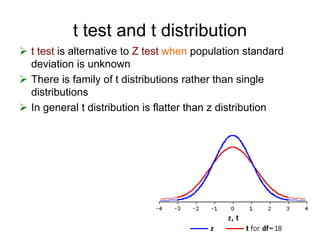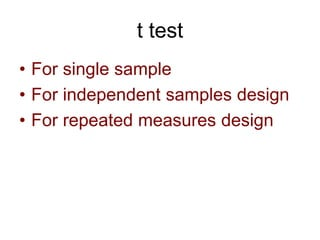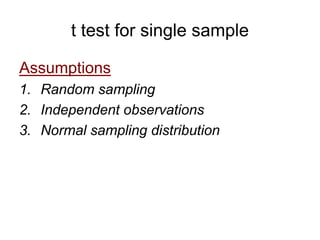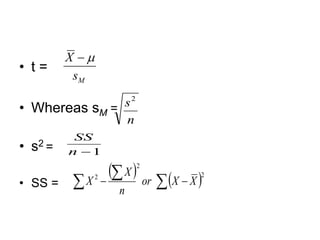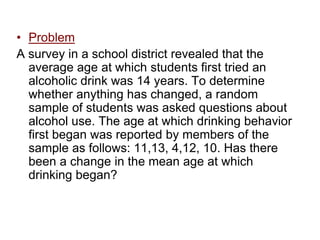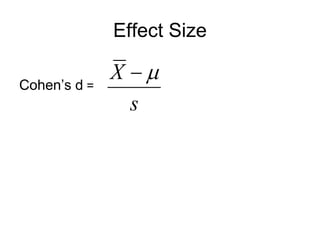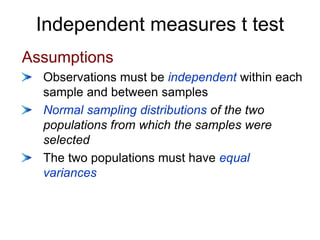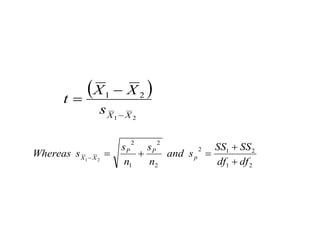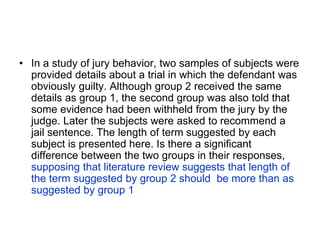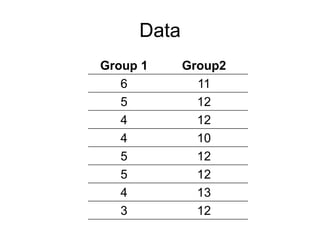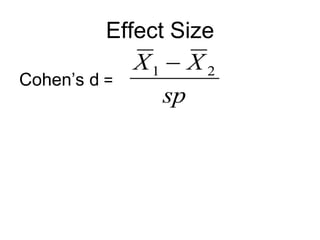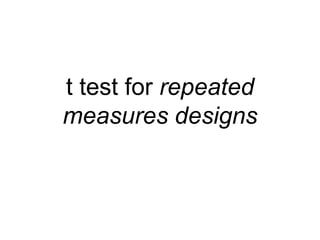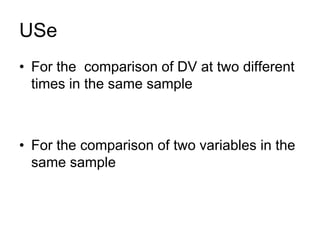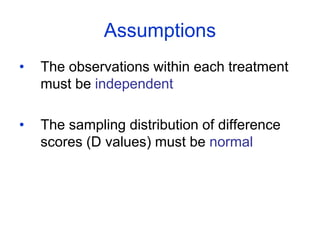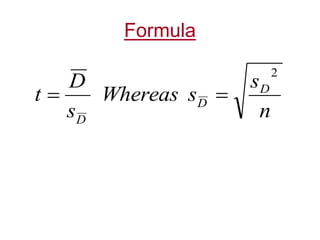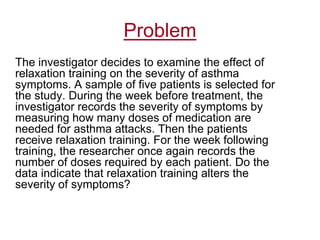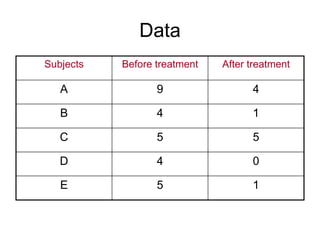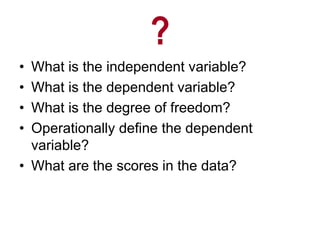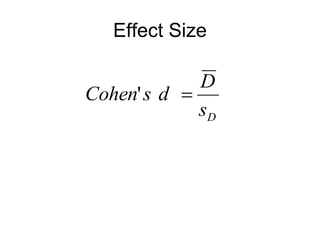1 of 19

### t test.ppt

1. t test and t distribution  t test is alternative to Z test when population standard deviation is unknown  There is family of t distributions rather than single distributions  In general t distribution is flatter than z distribution
2. t test • For single sample • For independent samples design • For repeated measures design
3. t test for single sample Assumptions 1. Random sampling 2. Independent observations 3. Normal sampling distribution
4. • t = • Whereas sM = • s2 = • SS = M s X   n s2 1  n SS    2 2 2      X X or n X X
5. • Problem A survey in a school district revealed that the average age at which students first tried an alcoholic drink was 14 years. To determine whether anything has changed, a random sample of students was asked questions about alcohol use. The age at which drinking behavior first began was reported by members of the sample as follows: 11,13, 4,12, 10. Has there been a change in the mean age at which drinking began?
6. Effect Size Cohen’s d = s X  
7. Independent measures t test Assumptions Observations must be independent within each sample and between samples Normal sampling distributions of the two populations from which the samples were selected The two populations must have equal variances
8.   2 1 2 1 X X s X X t    2 1 2 1 2 2 2 1 2 2 1 df df SS SS s and n s n s s Whereas p P P X X      
9. • In a study of jury behavior, two samples of subjects were provided details about a trial in which the defendant was obviously guilty. Although group 2 received the same details as group 1, the second group was also told that some evidence had been withheld from the jury by the judge. Later the subjects were asked to recommend a jail sentence. The length of term suggested by each subject is presented here. Is there a significant difference between the two groups in their responses, supposing that literature review suggests that length of the term suggested by group 2 should be more than as suggested by group 1
10. Data Group 1 Group2 6 11 5 12 4 12 4 10 5 12 5 12 4 13 3 12
11. Effect Size Cohen’s d = sp X X 2 1 
12. t test for repeated measures designs
13. USe • For the comparison of DV at two different times in the same sample • For the comparison of two variables in the same sample
14. Assumptions • The observations within each treatment must be independent • The sampling distribution of difference scores (D values) must be normal
15. Formula n s s Whereas s D t D D D 2  
16. Problem The investigator decides to examine the effect of relaxation training on the severity of asthma symptoms. A sample of five patients is selected for the study. During the week before treatment, the investigator records the severity of symptoms by measuring how many doses of medication are needed for asthma attacks. Then the patients receive relaxation training. For the week following training, the researcher once again records the number of doses required by each patient. Do the data indicate that relaxation training alters the severity of symptoms?
17. Data Subjects Before treatment After treatment A 9 4 B 4 1 C 5 5 D 4 0 E 5 1
18. ? • What is the independent variable? • What is the dependent variable? • What is the degree of freedom? • Operationally define the dependent variable? • What are the scores in the data?
19. Effect Size D s D d s Cohen  '